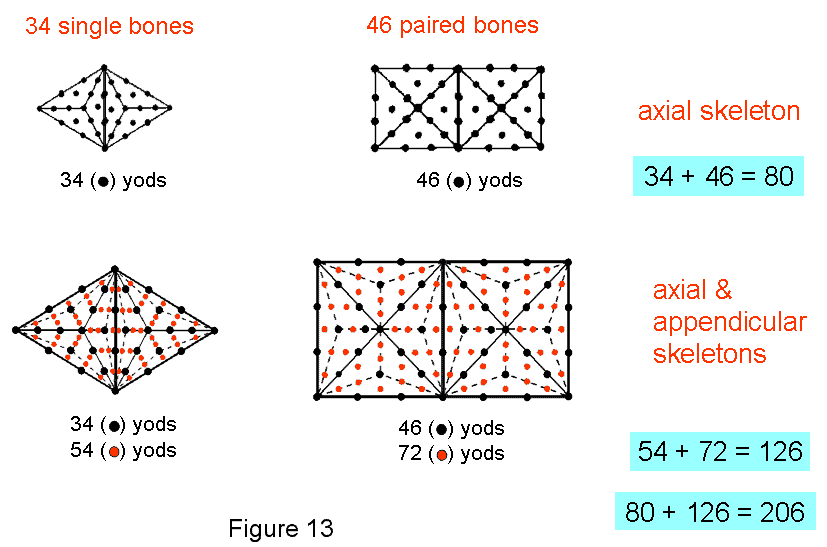Home

 << Previous    1...   7  8    10  11  ...15    Next >>

#9 The Type A & Type B triangles and squares embody the 206 bones of the human skeletonThe key to the encoding of scientific information in the inner Tree of Life is the transformation of the sectors of each polygon into tetractyses. Figure 13 shows that, when thus transformed, the pair of triangles joined at the ‘root edge’ contains 34 yods. Each yod symbolizes a single bone of the axial skeleton.  That this could be coincidental is made highly unlikely by the amazing fact that the next polygon — the square — embodies the number of pairs of bones in the axial skeleton!  Each square has 25 yods, that is, 21 yods outside their shared edge, so that the pair of them has (21+25=46) yods. The two sets of 23 yods symbolize the 23 pairs of bones. The 12 ribs on one side of the body are symbolized by the 12 yods inside the square that surround its centre. The six bones on either side of the face are denoted by the six hexagonal yods on the sides of each square. The two pairs of cranial bones are denoted by the two pairs of yods on the shared side of the squares, whilst the pair of three ossicles corresponds to the centre and two corners of each square. Transformation of the sectors of the pair of triangles and the pair of squares into Type A triangles yields the same number of yods as there are bones in the human skeleton! The triangle has 46 yods, of which 42 yods lie outside its edge shared with the other triangle. The pair of joined triangles contains (42+46=88) yods. The square has 61 yods, of which 57 yods lie outside its shared edge. The pair of joined squares contains (57+61=118) yods. There are (88+118=206) yods in the pair of triangles and the pair of squares. Whereas the transformation of the sectors of the pair of triangles and the pair of squares into single tetractyses generates 80 yods symbolizing the 80 bones of the axial skeleton, their transformation into three tetractyses generates 206 yods symbolizing the 206 bones of both skeletons. The additional number of yods (shown in red) is the number of bones in the appendicular skeleton! This remarkable result demonstrates the power of the tetractys to turn sacred geometry into numbers that are parameters of holistic systems like the human skeleton.

 << Previous    1...   7  8    10  11  ...15    Next >>

Home